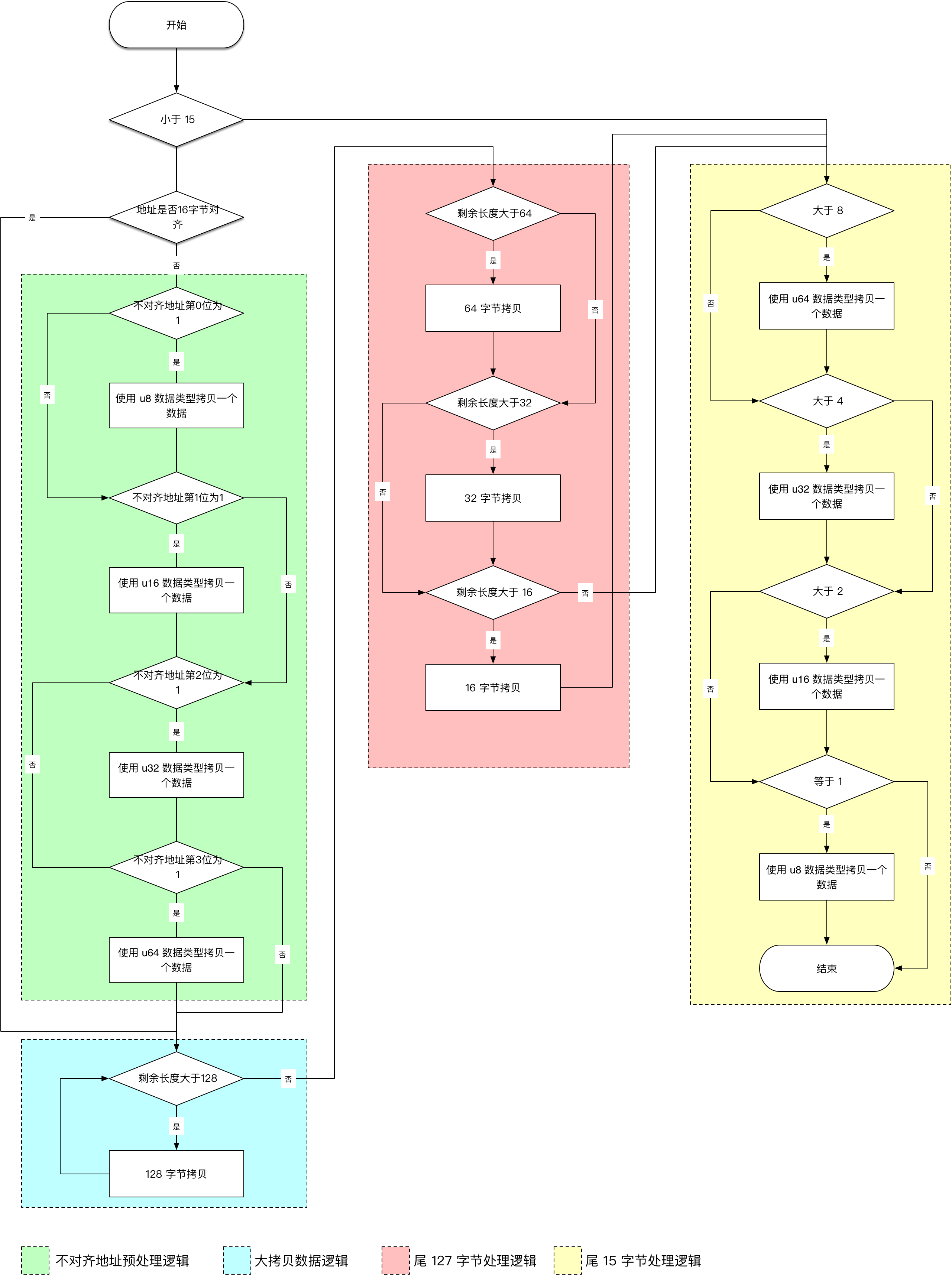# ARM64 的 memcpy 优化与实现

2018年7月2日 16.4k 次阅读 0 条评论 8 人点赞

### 如何优化 memcpy 函数

Linux 内核用到了许多方式来加强性能以及稳定性，本文探讨的 memcpy 的汇编实现方式就是其中的一种，memcpy 的性能是否强大，拷贝延迟是否足够低都直接影响着整个系统性能。通过对拷贝函数的理解可以加深对整个系统设计的一个理解，同时提升自身技术实力。

``````#ifndef __HAVE_ARCH_MEMCPY
/**
* memcpy - Copy one area of memory to another
* @dest: Where to copy to
* @src: Where to copy from
* @count: The size of the area.
*
* You should not use this function to access IO space, use memcpy_toio()
*/
void *memcpy(void *dest, const void *src, size_t count)
{
char *tmp = dest;
const char *s = src;

while (count--)
*tmp++ = *s++;
return dest;
}
EXPORT_SYMBOL(memcpy);
#endif
``````

``````void *memcpy(void *d, void *s, size_t count)
{
int i;
for (i = 0; i < count / sizeof(int64_t); i++) {
(int64_t *)d++ = (int64_t *)s++;
}
return d;
}
``````

``````void *memcpy(void *d, void *s, size_t count)
{
int i;
for (i = 0; i < count / sizeof(int) / 4; i++) {
(int *)d++ = (int *)s++;
(int *)d++ = (int *)s++;
(int *)d++ = (int *)s++;
(int *)d++ = (int *)s++;
}
return d;
}
``````

``````#include <arm_neon.h>

void *memcpy_128(void *dest, void *src, size_t count)
{
int i;
unsigned long *s = (unsigned long *)src;
unsigned long *d = (unsigned long *)dest;
for (i = 0; i < count / 64; i++) {
vst1q_u64(&d, vld1q_u64(&s));
vst1q_u64(&d, vld1q_u64(&s));
vst1q_u64(&d, vld1q_u64(&s));
vst1q_u64(&d, vld1q_u64(&s));
d += 8; s += 8;
}
return dest;
}
``````

### 当前 ARM64 构架的实现方式

``````ENTRY(__memcpy)
ENTRY(memcpy)
#include "copy_template.S"
ret
ENDPIPROC(memcpy)
ENDPROC(__memcpy)
``````

memcpy.S 直接 include 了一个 copy_template.S 的文件，其实就是直接贴上了这样的一份代码，这个 copy_template.S 不仅仅只是在 memcpy.S 中用到，在其他的类似 copy_to_user.S 和 copy_from_user.S 中也被包含。### memcpy 拷贝性能测试

``````typedef void *(*memcpy_t)(void *, void *, size_t);

void memcpy_speed_test(memcpy_t __memcpy, void *b1, void *b2)
{
int speed;
unsigned long now, j;
int i, count, max;

preempt_disable();

max = 0;
for (i = 0; i < 5; i++) {
j = jiffies;
count = 0;
while ((now = jiffies) == j)
cpu_relax();
while (time_before(jiffies, now + 1)) {
mb(); /* prevent loop optimzation */
__memcpy(bench_size, b1, b2);
mb();
count++;
mb();
}
if (count > max)
max = count;
}

preempt_enable();

speed = max * (HZ * bench_size / 1024);

printk(KERN_INFO "memcpy_test: %5d.%03d MB/sec\n", speed / 1000, speed % 1000);
}
``````

1. 其实还有一个优化的点就是注意 L1 Cache 的对齐，这个在汇编代码中有体现，C 语言版本就不提及 ↩︎